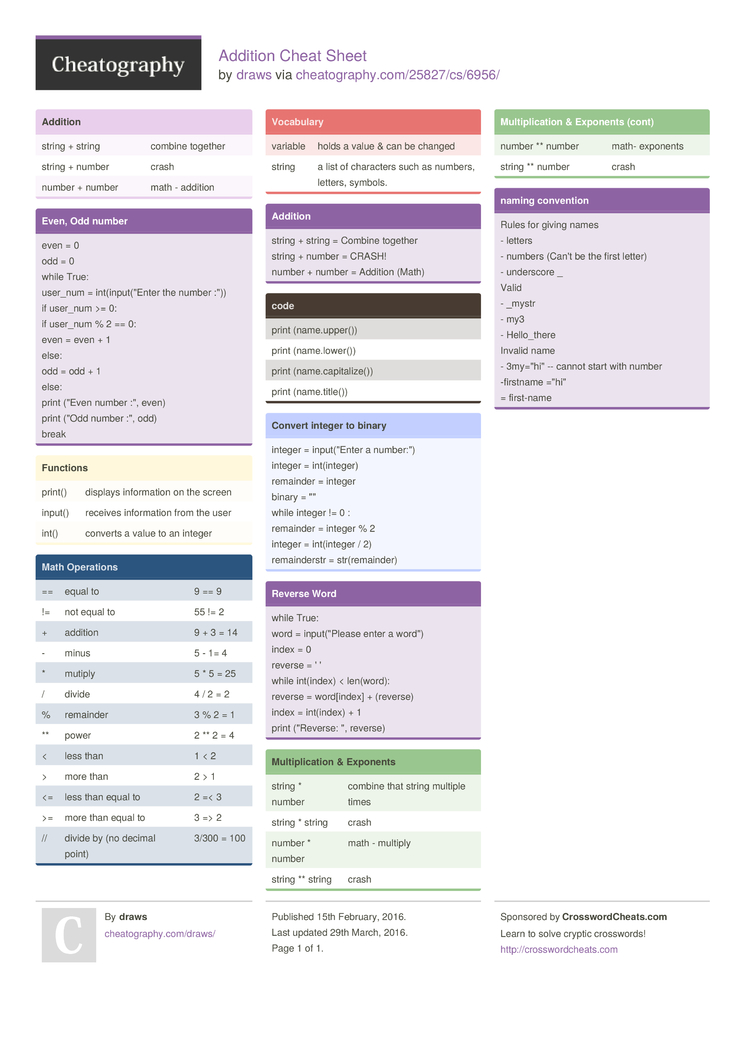Show Menu

# Addition Cheat Sheet by draws

### Addition

 string + string combine together string + number crash number + number math - addition

### Even, Odd number

 even = 0 odd = 0 while True: user_num = int(in­­pu­t­(­"­Enter the number :")) if user_num >= 0: if user_num % 2 == 0: even = even + 1 else: odd = odd + 1 else: print ("Even number :", even) print ("Odd number :", odd) break

### Functions

 print() displays inform­ation on the screen input() receives inform­ation from the user int() converts a value to an integer

### Math Operations

 == equal to 9 == 9 != not equal to 55 != 2 + addition 9 + 3 = 14 - minus 5 - 1= 4 * mutiply 5 * 5 = 25 / divide 4 / 2 = 2 % remainder 3 % 2 = 1 ** power 2 ** 2 = 4 < less than 1 < 2 > more than 2 > 1 <= less than equal to 2 =< 3 >= more than equal to 3 => 2 // divide by (no decimal point) 3/300 = 100

### Vocabulary

 variable holds a value & can be changed string a list of characters such as numbers, letters, symbols.

### Addition

 string + string = Combine together string + number = CRASH! number + number = Addition (Math)

### code

 print (name.u­pp­er()) print (name.l­ow­er()) print (name.c­ap­ita­lize()) print (name.t­it­le())

### Convert integer to binary

 integer = input(­"­Enter a number­:") integer = int(in­teger) remainder = integer binary = "­" while integer != 0 : remainder = integer % 2 integer = int(in­teger / 2) remain­derstr = str(re­mai­nder)

### Reverse Word

 while True: word = input(­­­"­­P­lease enter a word") index = 0 reverse = ' ' while int(index) < len(word): reverse = word[i­­­ndex] + (reverse) index = int(index) + 1 print ("Re­­­v­erse: ", reverse)

### Multip­lic­ation & Exponents

 string * number combine that string multiple times string * string crash number * number math - multiply string ** string crash number ** number math- exponents string ** number crash

### naming convention

 Rules for giving names - letters - numbers (Can't be the first letter) - underscore _ Valid - _mystr - my3 - Hello_­there Invalid name - 3my="hi­" -- cannot start with number -firstname ="hi­" = first-name

## Download the Addition Cheat Sheet1 Page
//media.cheatography.com/storage/thumb/draws_addition.750.jpg

PDF (recommended)

Alternative Downloads

## Comments

No comments yet. Add yours below!

## Add a Comment

Your Comment

Please enter your name.

Please enter your email address

Please enter your Comment.